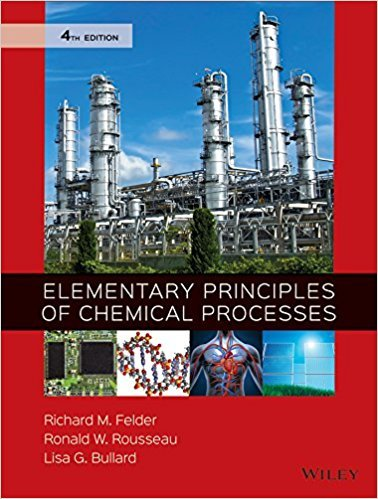×
×

# Solutions for Chapter A.2a: ITERATIVE SOLUTION OF NONLINEAR ALGEBRAIC EQUATIONS## Full solutions for Elementary Principles of Chemical Processes | 4th Edition

ISBN: 9780470616291Solutions for Chapter A.2a: ITERATIVE SOLUTION OF NONLINEAR ALGEBRAIC EQUATIONS

Solutions for Chapter A.2a
4 5 0 351 Reviews
31
3
##### ISBN: 9780470616291

This textbook survival guide was created for the textbook: Elementary Principles of Chemical Processes, edition: 4. Since 5 problems in chapter A.2a: ITERATIVE SOLUTION OF NONLINEAR ALGEBRAIC EQUATIONS have been answered, more than 42648 students have viewed full step-by-step solutions from this chapter. This expansive textbook survival guide covers the following chapters and their solutions. Chapter A.2a: ITERATIVE SOLUTION OF NONLINEAR ALGEBRAIC EQUATIONS includes 5 full step-by-step solutions. Elementary Principles of Chemical Processes was written by and is associated to the ISBN: 9780470616291.

Key Chemistry Terms and definitions covered in this textbook
• aldehydes.

Compounds with a carbonyl functional group and the general formula RCHO, where R is an H atom, an alkyl, or an aromatic group. (24.4)

• band

An array of closely spaced molecular orbitals occupying a discrete range of energy. (Section 12.4)

• bond order

The number of bonding electron pairs shared between two atoms, minus the number of antibonding electron pairs: bond order = (number of bonding electrons - number of antibonding electrons)/2. (Section 9.7)

• buffer solution.

A solution of (a) a weak acid or base and (b) its salt; both components must be present. The solution has the ability to resist changes in pH upon the addition of small amounts of either acid or base. (16.3)

• critical pressure

The pressure at which a gas at its critical temperature is converted to a liquid state. (Section 11.4)

• critical temperature

The highest temperature at which it is possible to convert the gaseous form of a substance to a liquid. The critical temperature increases with an increase in the magnitude of intermolecular forces. (Section 11.4)

• crystalline solid (crystal)

A solid whose internal arrangement of atoms, molecules, or ions possesses a regularly repeating pattern in any direction through the solid. (Section 12.2)

• Curved arrow

A symbol used to show the redistribution of valence electrons in resonance contributing structures or reactions, symbolizing movement of two electrons

• Dehydration

Elimination of water.

• Enamine

An unsaturated compound derived by the reaction of an aldehyde or ketone and a secondary amine followed by loss of H2O; R2C"CR!NR2

• hydrophobic

A nonpolar group that does not have favorable interactions with water.

• parts per billion (ppb)

The concentration of a solution in grams of solute per 109 (billion) grams of solution; equals micrograms of solute per liter of solution for aqueous solutions. (Section 13.4)

• Pauli exclusion principle

No more than two electrons may be present in an orbital. If two electrons are present, their spins must be paired

• polar reaction

A reaction that involves the participation of ions as reactants, intermediates, or products.

• probability density 1c22

A value that represents the probability that an electron will be found at a given point in space. Also called electron density. (Section 6.5)

• reverse osmosis

The process by which water molecules move under high pressure through a semipermeable membrane from the more concentrated to the less concentrated solution. (Section 18.4)

• SN1

A unimolecular nucleophilic substitution reaction.

• Tollens’ reagent

A solution prepared by dissolving Ag2O in aqueous ammonia; used for selective oxidation of an aldehyde to a carboxylic acid.

• unsymmetrical ether

An ether(R!O!R) where the two R groups are notidentical.

• Vibrational infrared region

A common type of spin-spin coupling involving the H atoms on two C atoms that are bonded to each other.

×